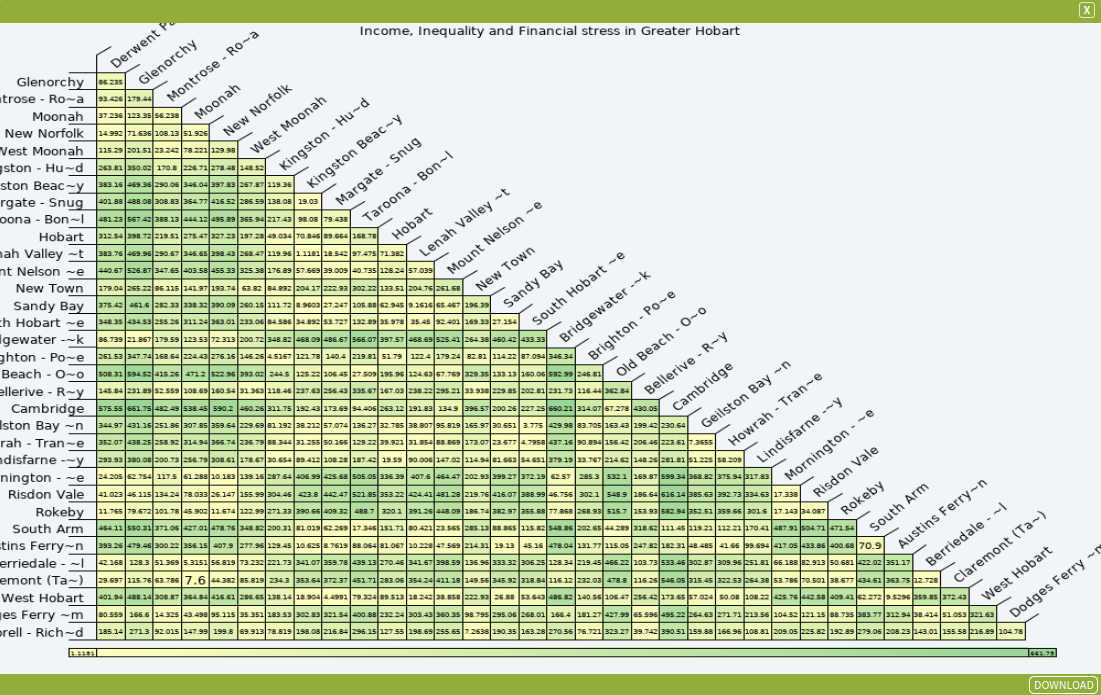# Hierarchical Clustering (Distance Matrix)

The Hierarchical Clustering Distance Matrix is a matrix (two-dimensional array) containing the distances, taken pairwise, of a set of points. This matrix will have a size of $N \times N$ where $N$ is the number of points, nodes or vertices.

The output of this is a graph which shows how similar each of the different areas are when taking into account a range of variables. Closeness to each other on the distance matrix suggests greater similarity.

### SET UP

To illustrate the use of the Hierarchical Clustering (Distance Matrix) tool, we will use a dataset on Income, Inequality and Financial Stress across the Greater Hobart area. To do this:

• Select Greater Hobart as your area.
• Select SA2 OECD Indicators: Income, Inequality and Financial Stress 2011 as your dataset, selecting all variables.

### Inputs

Once you have done this, open the Hierarchical Clustering (Distance Matrix) tool (Tools → Charts→ Hierarchical Clustering (Distance Matrix)) and enter the parameters listed below.

The parameters that need to be entered are:

• Dataset Input: Select a dataset that contains the variables of interest. Select SA2 OECD Indicators: Income, Inequality and Financial Stress 2011.
• Variables: A set of independent variables. Select the following variables:
• Median Disposable Income (Synthetic Data)
• Gini Coefficient (Synthetic Data)
• Poverty Rate (Synthetic Data)
• % Can’t afford a night out (Synthetic Data)
• Distance Metric: Distance measure to be used. Select euclidean.
• euclidean: “ordinary” straight-line distance between two points in Euclidean space.
• maximum: greatest distance along any coordinate dimension, also known as chessboard distance.
• manhattan: the distance between two points measured along axes at right angles.
• canberra: a measure of similarity and dissimilarity between groups.
• binary: measures the minimum number of substitutions required to change one string into the other, or the minimum number of errors that could have transformed one string into the other.
• minkowski: is a metric in a normed vector space which can be considered as a generalization of both the Euclidean distance and the Manhattan distance.
• Cluster Metric: The agglomeration method (linkage rule) to be used. It is important to note that in every method used, the analysis is processed as a complete-link case. Select complete.
• ward: calculates the increase in the error sum of squares (ESS) after fusing two clusters.
• single: the two closest points from each cluster
• complete: the two furthest points from each cluster
• average: the average of the cluster’s distances is taken whilst compensating for the number of points in that cluster
• mcquitty: the average of the cluster’s distances is taken, not considering the number of points in that cluster.
• median: the inter-cluster median point
• centroid: the inter-cluster mid-point
• Observation Labels: A variable whose values are to be used as labels for each case. Select SA2 Name.
• Chart Title: A title for your Hierarchical Clustering Dendrogram. Type Income, Inequality and Financial stress in Greater Hobart.
• Greyscale: Specify whether you would like your graph to be grey-scale (checked) or colour (unchecked). Untick this box.Note: Please see the documentation of Cluster Analysis (Hierarchical) for further details.

Once you have selected your parameters, click Run Tool.

### Outputs

Once you have run the tool, click the Display Output button which appears in the pop-up dialogue box. This should open up a chart tool looking like the one shown below.The output shows the distance between each Hobart SA2. The smaller the number, the closer they are in relation to the variables chosen. We can see that Kingston Beach is close to Claremont, as it has a value of 18.904, compared to Bellerive and Bridgewater that have a high value of 660.21 indicating that they are considered further apart.

### Looking for Spatial Data?

You can browse the AURIN Data Discovery:### How can you Create Impact?